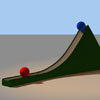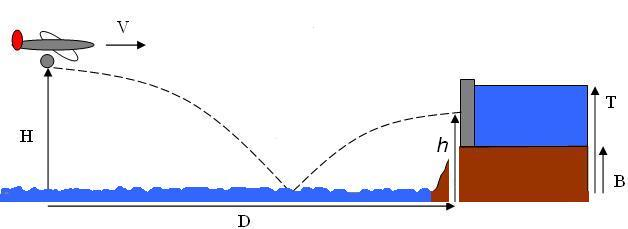#### You may also like### Whoosh

A ball whooshes down a slide and hits another ball which flies off the slide horizontally as a projectile. How far does it go?

# Dam Busters 2

##### Age 16 to 18 Challenge Level:

In the problem Dam Busters 1 we looked at targetting a dam with a direct hit. In that case the plane could only just get close enough to the dam to destroy its target. The dam is destroyed if the bomb strikes above the rocky outcrop of height $B$.

A scientist has suggested developing a bomb which will bounce on water when travelling transverse to the water surface at high speed. It has been suggested that this bouncing bomb will enable the target to be struck more centrally whilst releasing it from a greater distance.Several scientists have tried to calculate the height h of the bouncing bomb above sea level at the point at which it will be level with the wall after the first bounce. The scientists agree on the overall general form of the equation but cannot agree on some of the signs and which way around some of the factors should go.

Can you decide whether each of the signs in the equation should be 'plus one' or 'minus one' (they can be different from each other)? $$h = -\frac{1}{2}g^{\pm 1}\left[\left(\frac{V}{D}\right)^{\pm 1}-\sqrt{X}\right]^2 \pm eg\sqrt{X}\left[\left(\frac{V}{D}\right)^{\pm 1}-\sqrt{X}\right] \;,\quad\quad X = \left(\frac{2H}{g}\right)^{\pm 1}$$

In the equation, $e$ is the coefficient of restitution for the bomb striking the water. $e$ is dimensionless and positive. You do not need to derive the equation, only to decide which configurations would give rise to a sensible equation from a physical point of view.

Now that you have chosen the correct equation, (and checked that it is correct!) explore the heights and distances $H$ and $D$ which will enable a strike on target when the coefficient of restitution is $0.8$. The bomber must fly below $200\mathrm{m}$ and release the bomb at a minimum distance of $1 \mathrm{km}$ from the target. The bomber can fly at up to $800\textrm{ km per hour}$.

What is the minimum value of the coefficient of restitution for which this bombing strategy will work in this situation?

Extension: Derive this equation used in the calculations

NOTES AND BACKGROUND
Bouncing bombs were indeed used in World War II to destroy dams.

In 1941 Barnes Wallis, a scientist working for a British aircraft company was commissioned to investigate ways to halt the German war machine. He concluded that destruction of dams in the industrial Ruhr region would be devastating to German production. An 'Air Attack on the Dams Committee' was formed to try to find targets.Of course, the dams were immensely strong and very heavily defended. Barnes realised that the only way to destroy a dam was for a bomb to explode at its foot, which was protected by torpedo nets. The committee believed that this problem was technologically intractable.

Thinking out of the box Barnes invented the bouncing bomb. In the final attack the plane had to fly at 220 miles per hour, at a height of 60 feet above the water and release the bomb from exactly 425 yards.